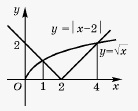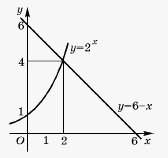# Graphical Solving of Equations

On practice it is often useful to use graphical method to solve equations. The essence of this method is in following: to solve equation f(x)=0 we draw graph of the function y=f(x) and find x-intercepts; these points will be roots of the equation.

For example, graph of the function y=-0.5x^2-x+4 intersects x-axis at points (-4;0) and (2;0), therefore equation -0.5x^2-x+4=0 has two roots: x_1=-4 and x_2=2.

Graph of the function y=x^2-4x+5 doesn't intersect x-axis, therefore, equation x^2-4x+5=0 doesn't have roots.

Often, equation f(x)=0 is replaced with equivalent equation g(x)=h(x), then we draw graphs of the functions y=g(x) and y=h(x) (if it is easier, then drawing of y=f(x)) and find x-coordinates of points of intersections of the graphs.

Example 1. Solve graphically sqrt(x)=|x-2|.Let's draw graphs of the functions y=sqrt(x) and y=|x-2| (see figure). They intersect at points with coordinates x_1=1,x_2=4. These are two roots of the given equation.

With graphical method for solving equations connected some analytical methods, that are based on using different properties of function f(x) and g(x).

Let's formulate two facts that are base for these methods.

Fact 1. If one of the functions f(x), g(x) is decreasing, and another is increasing in the domain of the equation f(x)=g(x), then this equation either has no roots, or has only one root.

Fact 2. Let function f(x) is increasing (decreasing), i.e. is monotone, on interval X, and number a is any of values, that function f(x) can take on this interval. Then equation f(x)=a has only one root on the interval X.

Example 2. Solve graphically equation 2^x=6-x.It can be easily seen that x=2 is root of the equation. Since function y=2^x is increasing, and function y=6-x is decreasing then there are no other roots of given equation.